# Inconsistency between tables and flow variables

Dear Knimers,

there are some inconsistencies between the behavior of tables and that of flow variables. One well-known one is that a table can contain missing values but a flow variable cannot; the Table Row To Variable and Table Row To Variable Loop Start force the user to replace the missing value with a non-missing default value, to fail the execution, or to omit the variable entirely. I guess that the reason behind this is that, for example, the Java Double class does not support missing values and making a special DoubleKNIME class would not be worth the added complexity. Similarly, allowing the variable to be “null” would probably lead to a software engineering nightmare.

Another inconsistency is that when evaluating 0/0 in a Math Formula node, the result is a missing value. In the Math Formula (Variable) node, the result is NaN. When this is converted to a table with a Variable to Table Column node, the result is a column filled with NaNs. I.e., the result of identical expressions is different depending on whether they are performed on variables or tables.

Here is another inconsistency that I came across today. Suppose I have a table: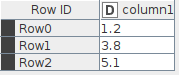and I want to convert it to integers using the Math Formula node: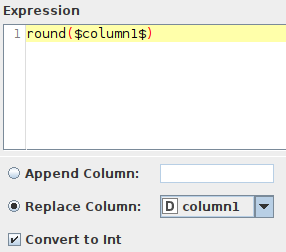The result is exactly what I would expect, a table where original column has been replaced: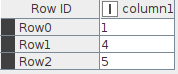Let’s try this with a flow variable: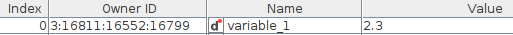and a Math Formula (Variable) node: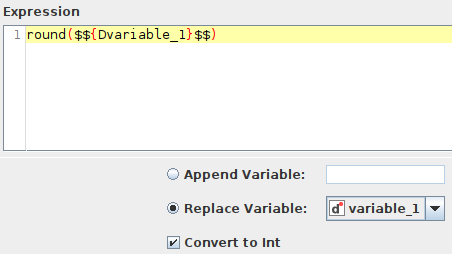The result is TWO flow variables with the same name but different types, even though “Replace” is checked.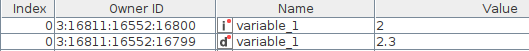I think the “Replace Variable” option should be greyed out when the “Convert to Int” option is checked.

Best,
Aswin

1 Like

Hi @Aswin,

Thank you very much for your message. Let me comment on the different topics you brought up.

Issue #2: Quoting from the Math Formula documentation

When any of the used columns contains a missing value, the result is missing, just like when the result would be NaN, infinite value, or outside of the 32 bit signed integer range when that is requested

You are right, however, that this is inconsistent with the result produced by other nodes such as the Math Formula (Variable) and the Column Expression node, both able to output NaN and Infinite values. I have opened a ticket for our developers (internal reference AP-19994) to look into that and will keep you posted.

Issue #3: This is already documented (AP-16885). I added a +1 from you to the ticket.

Once again, many thanks for reporting this.
Have a great day,
Emilio

2 Likes

Thank you Emilio! I am just thinking how awesome it would be if any type of information that could be contained in a table cell could also go into a flow variable; no matter whether it is a String, Double, PNG image or molecular structure. It would remove a lot of workflow engineering pitfalls and complexity. One can dream….